# Ncert Class 6 Math Fractions Exercise 7.5

Fractions Class 6 Ex. 7.5

New Ncert Class 6, Chapter 7 Math Free Solution.

Exercise 7.5

Question 1 :- Write; these fractions appropriately as additions or subtractions.

(a)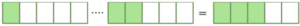(b)(c)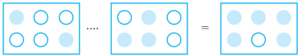Solution 1:-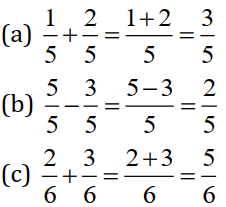Question 2. Solve: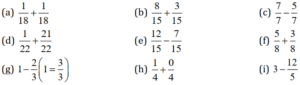Solution 2:-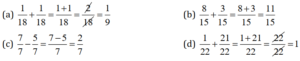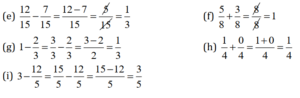Question 3 :- Shubham; painted 2/3 of the wall-space in his room. His sister Madhavi; helped and painted 1/3 of the wall-space. How much did they paint-together?

Solution 3:-
2/3 of the wall was painted by Shubham.
1/3 of the wall was painted by Madhavi.
Fraction of wall painted by Shubham and Madhavi = 2/3+ 1/3 = (2+1)/3 = 3/3 = 1

Thus the fraction of wall painted by both = 1 ( The complete wall )

Question 4 :- Fill in the missing-fractions.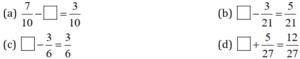Solution 4:-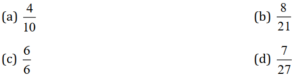Question 5 :- Javed was given 5/7; of a basket of oranges. What fraction of orange was left in the basket?
Solution 5:-
Fraction of basket of oranges = 5/7
Fraction of basket as a whole can be considered as 1.

So; Fraction of basket of oranges left = 1 – 5/7 = (7-5)/7 = 2/7

Thus, the required fraction = 2/7 .